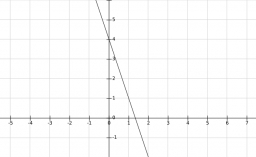# Intersections 62534

The equation of the linear function is: y = -3x + 4
(a) determine the intersections with the axes
sketch chart

b) for which x applies
f (x) = - 1

c) for which x applies
f (x) = 0

d) for which y applies
f (-1/2) = y

x0 =  1.3333
y0 =  0
x1 =  0
y1 =  4
x2 =  1.6667
x3 =  1.3333
y4 =  5.5

### Step-by-step explanation:Did you find an error or inaccuracy? Feel free to write us. Thank you!